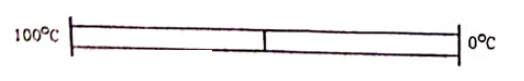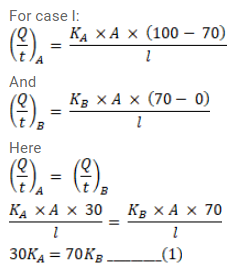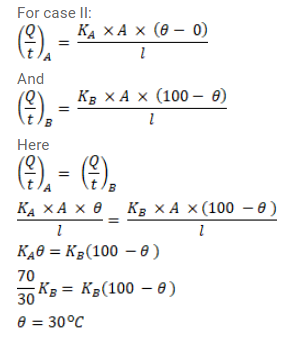# The two rods shown in figure have identical geometrical dimensions.Question:

The two rods shown in figure have identical geometrical dimensions. They are in contact with two heat baths at temperatures $100^{\circ} \mathrm{C}$ and $0^{\circ} \mathrm{C}$ The temperature of the junction is $70^{\circ} \mathrm{C}$. Find the temperature of the junction if the rods are interchanged.Solution: